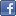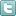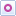EE Times-India > Sensors/MEMS

Sensors/MEMS# Utilising FETS for sensor applications (Part 2)

Posted: 10 Jul 2015Print Version

Keywords:op amps  FET  photodiode preamp  transresistance amplifiers  hydrophone

In Part 1, we discussed bipolar input stage noise, piezoelectric pre-amps and charge amplifiers.

Photodiode preamps
In some JFET op amps such as the AD743, the input capacitance is in the order of 18 to 20 pF. In comparison, with an LSK489 dual FET, the input capacitance is in the order of 3 pF, which will be suitable for low noise photodiode applications. In this section we will see why it is important to have low equivalent input noise and low input capacitance in a photodiode preamp.

A simple photodiode is shown in figure 8, which uses an op amp.Figure 8: A simple photodiode transresistance amplifier.

In the photodiode amplifier above, when light is shined onto the photodiode, current is generated by the photodiode, PD1. As configured with the cathode of PD1 connected to the (-) input terminal of U1, Vout generates a positive voltage proportional to the amount of light into the photodiode. Also shown in figure 8 are the equivalent capacitances from the photodiode, Cpd, and (-) input terminal, Cin(-), which are connected in parallel. To minimise Cpd, the photodiode capacitance, the anode of PD1 is connected to the minus 12V power supply for maximum reverse bias to lower its junction capacitance. For example, if a BPV10 photodiode is used, Cpd is about 2.7 pF at 12V reverse bias. At a lower reverse bias voltage such as 1V, Cpd is about 7 pf.

For low noise considerations, these two capacitances, Cpd and Cin(-), should be low as possible. The reason is that the equivalent input noise density voltage, Vnoise_input of the op amp will be amplified in the following manner at Vout, neglecting any noise current from the photodiode:

Vout_noise for a bandwidth of 1Hz = (Vnoise_input) √(1+ωRFCt)2 + √4kTRF (1)

Where
ω = 2πf, RF = feedback resistor, k = 1.38 x10-23J per degrees Kelvin, T = 298 degrees Kelvin

Ct = Cpd||Cin(-) = total capacitance at the (-) input terminal, and
Ct = Cpd + Cin(-)

√4kTRF = thermal noise voltage of the feedback resistor RF for a bandwidth of 1Hz.

As we can see from the equation above, the output noise, Vout_noise, goes higher if Ct is increased.

In designing a low noise transresistance preamps the goals are to:
1) Minimum equivalent input noise voltage. Equation (1) above shows that the output noise voltage is dependent on the equivalent input noise voltage, Vnoise_input.
2) Minimise noise current from the (-) input because the noise current at the input will form a noise voltage across the feedback resistor. Generally, a JFET is desirable for the (-) input because of its low gate noise current.
3) Minimise the capacitance from the (-) input to ground. The equation (1) shows that more noise is generated at the output when the capacitance, Ct = Cpd + Cin(-), at the (-) input terminal is increased.
4) Use as large value RF as possible. At first glance, it would appear increasing the resistance in RF would increase the output noise because of the resistor's thermal noise. This is true but the signal amplification from the photodiode is increased more so that results in a net increase in signal to noise ratio when RF is increased in value. For example, doubling the value in RF increases the resistor noise from RF by √2 = 1.41 while increasing the photodiode signal output voltage by 2. Thus, there is a net gain of √2 or + 3 dB, in terms of signal to noise ratio in this example.

Lowering capacitance of the op amp
In figure 8, the typical input capacitance, Cin(-) at the (-) input of an FET op amp is about 18 pf. To lower the capacitance of the op amp, a low capacitance and low noise JFET is used as a buffer or source follower to the (-) input (figure 9).Figure 9: A low capacitance JFET added to an op amp circuit to lower input capacitance and reduce noise.

 Related Articles Editor's Choice
Comment on "Utilising FETS for sensor applicatio..."

Top Ranked Articles

WebinarsVisit Asia Webinars to learn about the latest in technology and get practical design tips.

Search EE Times India
Services

Go to topConnect on FacebookFollow us on TwitterFollow us on Orkut
﻿